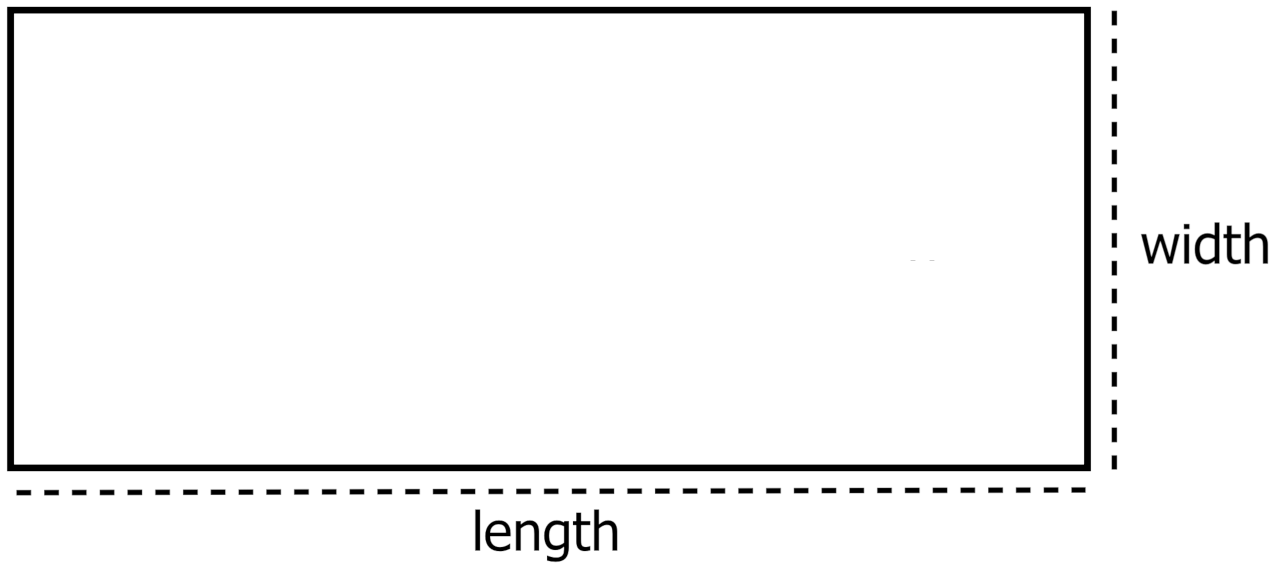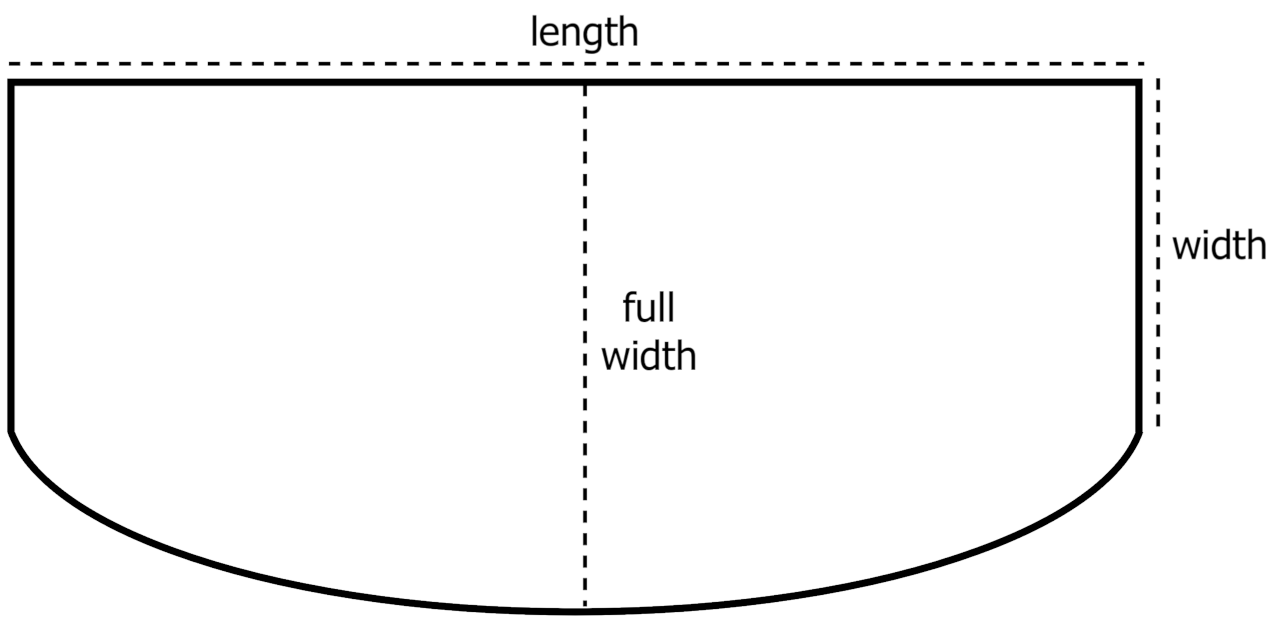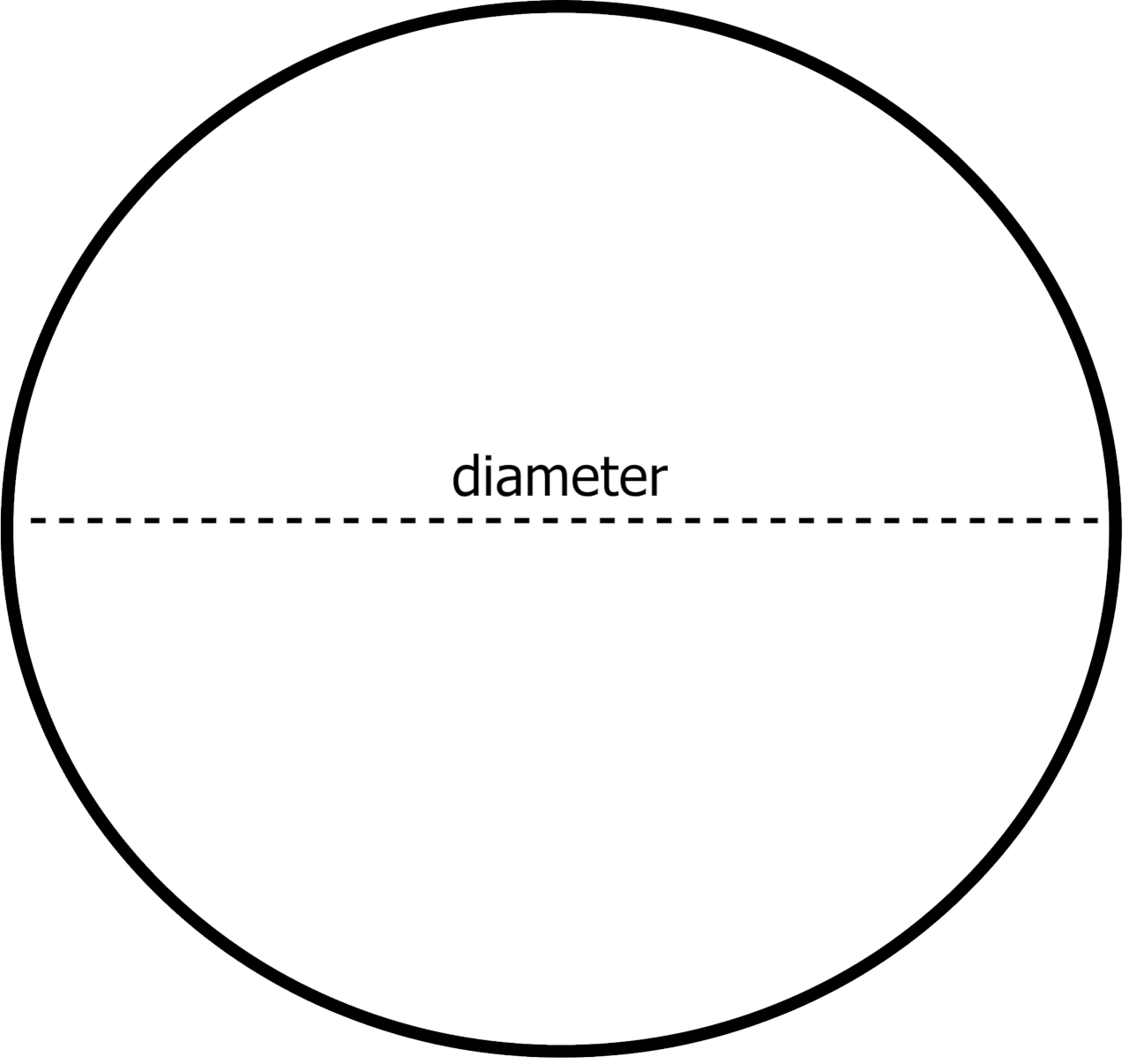# Aquarium Tank Volume Calculator

Calculate the volume of water in a fish tank by selecting the tank shape and entering its dimensions.

## Tank Volume Estimate:

gallons

liters
Learn how we calculated this below

## How to Estimate Aquarium Water Volume

You can estimate the amount of water that a tank can hold by using the volume formula for the shape of your tank. The process is similar to calculating tank volume for other tanks, but the formulas are a bit different for different aquarium shapes.

You’ll need a different formula to find the volume for rectangular, bow front, cylindrical, and other aquarium shapes.

### Tank Volume Formulas

See the volume formulas for various aquarium fish tank styles below.

#### Rectangular Tank Formula

tank volume = l × w × h

l = tank length
w = tank width
h = tank height#### Bow Front Tank Formula

square area = l × w
elliptical area = π × (l ÷ 2) × (fw – w) ÷ 2
tank volume = (square area + elliptical area) × h

l = tank length
w = tank width
fw = tank full width
h = tank height#### Cylinder Tank Formula

r = d ÷ 2
tank volume = π × r2 × h

d = tank diameter
h = tank heightFind even more volume formulas on our volume calculator.

### Solve the Formula

Once you have the appropriate volume formula for the tank, measure the required dimensions and solve the equation. For instance, if the aquarium is a standard rectangle, use a tape measure to measure the length, width, and height in inches. Then, enter the dimensions measured into the formula and solve.

### Convert to Gallons or Liters

The result of the formula will be the volume in cubic inches, or whatever unit was used in the initial measurements. The easiest way is to convert this to gallons or liters using a conversion calculator. You can also divide the number of cubic inches by 231 to get the volume in gallons.

For example, let’s calculate the volume of a rectangular tank that is 20″ long, 12″ wide, and 10″ high.

Start by calculating the volume in cubic inches.

tank volume = 20 × 12 × 10
tank volume = 2400 cu in

Convert cubic inches to gallons.

gallons = 2400 cu in ÷ 231
tank volume = 10.39 gallons

The calculator above will simplify this process, simply enter the dimensions in the fields and it will estimate the water in gallons and liters.

Curious how much your filled aquarium weighs? Try our aquarium weight calculator.